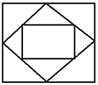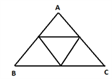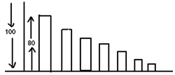# Infinite Geometric Progression

#### What is an infinite GP?

Consider the following GP 2, 1, 1/2 ………..

This GP goes on to infinity and is referred to as an infinite GP The sum of an infinite GP is denoted as

S8 = a/((1-r) ) where r < 1 Where “a” is the first term and “r” is the common ratio.

The sum to infinity of the example above is 2/((1-1/2) ) = 4

Let us look at some application based questions on infinite GP

### Illustrations:

24. A square is drawn by joining the midpoints of the sides of a given square, a third square drawn inside the second square in the same way and this process continues indefinitely, if the side of the first square is 12 cm, what is the sum of areas of all the squares?a) 1024 b) 512 c) 288 d) 1200

Solution:Area of the first square = 144

Area of the 2nd square = 72

Area of the third square = 36

i.e.It will be an infinite GP 144, 72, 36, 18, 9……… with first term, a = 144 and

common ratio, r = 1/2 Sum to infinity = a/(1-r)= 288 So sum of areas of all the squares = 288 sq. units

This concept can be extended to triangles as well. Try getting the answer on your own.

25. The mid points of a triangle are joined. Forming a second triangle, a third triangle is formed joining the midpoints of the second and the process continued infinitely. If the area of the first triangle is 24 units, then find the sum of areas of all triangles.

a) 26 b) 28 c) 32 d) 3626. After striking the floor, a ball rebounds to 4/5th of the height from which it has fallen. Find the total distance it travels before coming to rest if it is dropped from 100 meters. a) 400 b) 500 c) 700 d) 900.

Solution:Here the ball is dropped from a height of 100 m. Then it will rebound back up to 80m (but you will have to consider distance travelled in both the directions, up and down)

So the required GP will be 100 + 2 [80 + (0.8 × 80) + (0.8 × 0.8 × 80) + ……..]

Part inside the square bracket is an infinite GP with first term, a = 80 and common ratio, r = 0.8

Sum to infinity of this GP = 80/(1-0.8) = 400

Required answer = 100 + 2 × 400 = 900